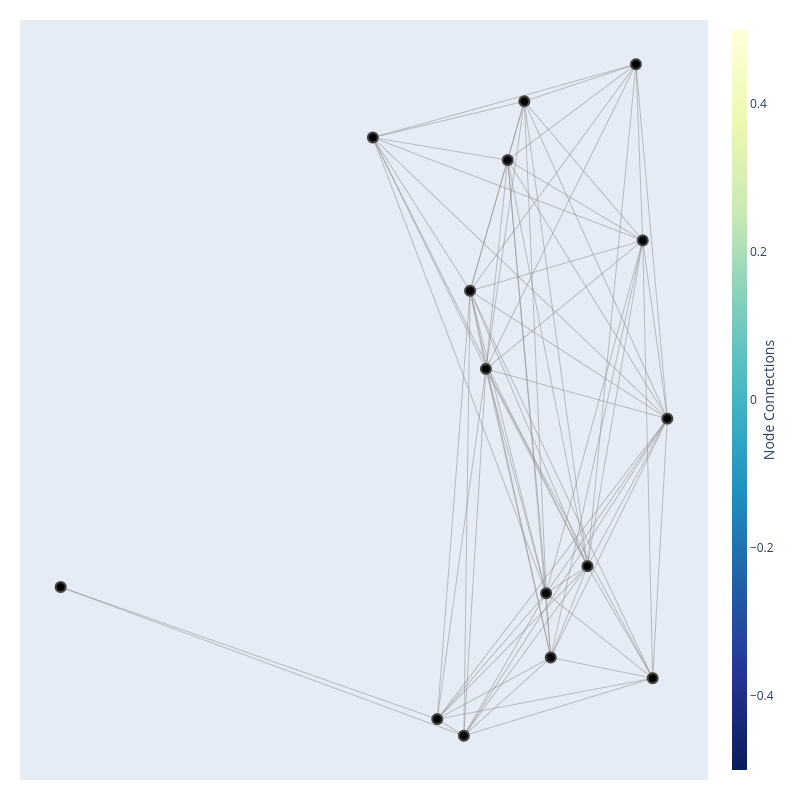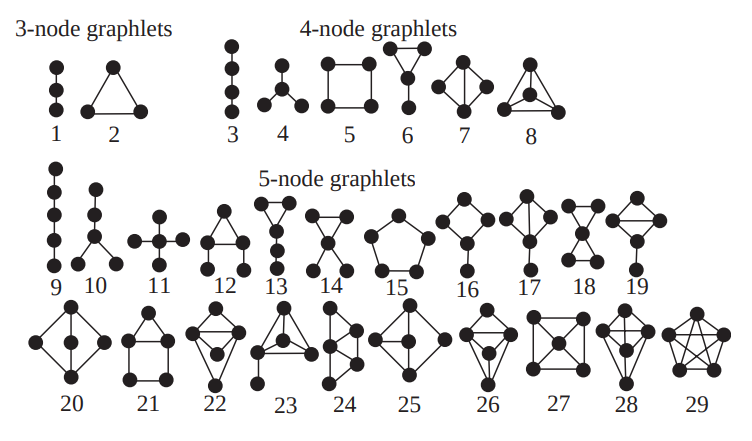# .css-fhxb3m{display:-webkit-box;display:-webkit-flex;display:-ms-flexbox;display:flex;-webkit-flex-direction:row;-ms-flex-direction:row;flex-direction:row;-webkit-align-items:center;-webkit-box-align:center;-ms-flex-align:center;align-items:center;}.css-9a49z0{margin-right:0.5rem;height:2.5rem;width:2.5rem;-webkit-flex-shrink:0;-ms-flex-negative:0;flex-shrink:0;overflow:hidden;border-radius:9999px;}Aadi's Blog

Follow

Follow# Node Features for Graph Machine Learning

·Jul 8, 2022·

A graph in the context of mathematics is was almost every other field refers to as a network. It consists a series of nodes connected by edges, both of which can contain meta- information, or what we refer to as features.For example, a graph can describe power-stations across some geographic area; Each station could have features describing its maximum output, current output and current demand as its node features. The edges could describe the energy flowing to distribution stations, or other power-stations in times of high demand. An interesting application may be to forecast the required power output for any station or for the consumption of any distribution station at a given point in time. The benefit of using a graph-based approach in this case is an implicit way to capture the dependencies between stations that deliver power to similar geographic areas, where each node is "aware" of other nodes at given time-steps, and therefore can adjust itself in relation to other nodes for more efficient energy use.

See here for an interesting application similar to what was described above

Before we get ahead of ourselves, we should take a step back and think about how we go about making predictions on a graph. Supervised machine learning uses features constructed from our data to establish (hopefully) pronounced similarities and differences in the input data that may not have been immediately obvious. In order to do this, we take our data (graph with node and edge features) and construct more appropriate features that can more easily be digested by a traditional machine-learning model.

There are three (traditional) ways of going about this, we can construct features on the nodes, on the edges or on the entire graph at a time. In this post, I will go through node features, where it's used, and some high-level intuition about each.

# Node Importance

Identifying important vertices in a graph is an interesting problem that usually requires some the development of some node-level embedding.

## Node Degree

Caveat: this technically is a type of centrality, but I keep it separate as its sometimes referred to as a node "feature"

The most obvious way to create a smart feature describing nodes u in a graph V is to take a count of the node degree, or the number of edges leaving or entering a node (or just the number of edges if the graph is un-directed).

$$d u = \sum {u \in V}\textbf{A}[u, v]$$

As intuitive as this is, there are some drawbacks; Counting the number of edges of a given node only accounts for the nodes directly local neighborhood, and it ignores other node features (such as how important a neighbor may be) that may contribute to informative statistics from the graph. Lastly, the importance of a given node should (I think?) depend on the importance of my neighbors. For example, in a given social network, I think if you had a direct edge to the President of the US, your "importance" should be more heavily weighted.

## Node Centrality

Node centrality aims to improve upon vanilla node degree, by addressing its main shortcoming of not accounting for neighbors' importance. Additionally the idea of "centrality" encompasses a range of different methods, few of which I go through here

### Betweenness Centrality

This measure accounts for the number of shortest-paths to a given node. Going back to the social-media example, if lots of my friends are directly connected to an important person, then that makes them more important, and therefore (assuming it's actually a lot of friends), I may then be weighted as a more "important" person.

This measure counts the number of shortest paths which go through a given node, therefore making it more "central" to the graph. Another way is to think of junctions in a city as nodes, and edges as roads; if you have to pass through the main junction to reach most of the other junctions, it's highly likely that it is indeed important.

For completeness, here's how we calculate betweenness centrality of a node v:

$$C(v) = \sum_{\text{all node pairs in graph}}\frac{\text{Number of shortest paths between two nodes that pass through node v}}{\text{Number of shortest path between two nodes}}$$

### Closeness Centrality

This measure counts how far away a node is from every other node. The idea is, if a node is further from every other node, it is less important (note the concept of "important" here can be flipped if we rank important-ness in rarity, or if we want to find outlier nodes).

For a given node v, this measure is taken as the sum of 1 over the number of edges in every shortest path to every other node.

$$C(v) = \frac{1}{\sum_{\text{every other node}}\text{(Number of edges in shortest path)}}$$

## Eigenvector Similarity

This is an interesting way of simply updating node importance based on neighbor importance, and requires a bit more background. We represent a graph as an adjacency matrix (other ways are as an edge list or adjacency set), which is square and has the number of rows/columns equal to the number of nodes in a graph. A non-zero entry indicates connection between two nodes. A node's eigenvector similarity is defined as following:

$$eu = \frac{1}{\lambda}\sum{u\in V}\bold{A}[u, v]e_v$$

If we rewrite the above in vector notation, with e as a vector of node centralities:

$$\lambda\bold{e} = \bold{Ae}$$

The above is of the form of the eigenvector-eigenvalue decomposition (see Section 4.4 of this free online book for a great breakdown).

A given view of this measure is that it ranks the likelihood that a node is visited on a random walk of infinite length on the graph.

The part to note here is that, assuming we require positive centrality values, there are theorem which allow us to solve this iteratively computationally.

# Structure Based Features

## Clustering Coefficient

This is taken as the fraction of existing connection among a node's neighbors divided by the total number of possible connections.

It corresponds to the probability that two nearest neighbors of a node are connected with each other. In another view: clustering coefficient measures the proportion of closed triangles in a nodes local neighbor hood, giving an idea of how tightly knit a node's neighborhood may be.

There are many variations of this metric, of which a popular version called the local is computed as follows:

$$c_v = \frac{\text{Number of edges between neighbors of node }v}{\text{Number of pairs of nodes in neighborhood}}$$

## Graphlet Degree Vector

A graphlet is a collection of nodes, that can contribute to a subgraph of a given network. This counts the number of graphlets rooted at each given node (up to a given size or of a given type). The graphlet degree vector is a vertical column of how many graphlets of a particular count appears rooted at a given node.From Pruzi et. al. 2004

For example, to get the graphlet degree vector of a node for graphlets up to five-nodes, every node would have a vertical vector of 73 values, representing the various types of graphlets, where each number represents the number of a given 5-node graphlet which appears rooted at a given node. An interesting note is that the graphlet-frequency vector is fairly robust to random node addition/deletion and rewiring. This may be beneficial for classification tasks, but may not be favorable for outlier-analysis tasks. Additionally, this measure has been used as a basis for graph comparison tasks, in fairly recent papers such as Graphlet-based Characterization of Directed Networks

See here for a more thorough application of the graphlet-degree vector.